# Financial Planning with formulas

Country: Universal  | Accounting type: Double-Entry Accounting  |  Category: Education

Bar Luca's

Basic Currency: .

Double-entry

Accounts
Group Account Description Gr
BALANCE SHEET
ASSETS
CASH Cash on hand 1
BANK Bank account 1
CUSTOMERS Customers account 1
FURNITURE Furniture 1
INVENTORY Inventory 1
1   TOTAL ASSETS 00

LIABILITIES AND EQUITY
SUPPLIERS Suppliers 2
LOAN Loan 2
EQUITY Owner equity 2
PL   Profit(-) or loss (+) from current year 2
2   TOTAL LIABILITIES AND EQUITY 00

PROFIT AND LOSS STATEMENT
INCOME
SALES Income from sales 4
INTINC Interest income 4
4   TOTAL INCOME 02

EXPENSES
PERSON Personnel expenses 3
GOODS Cost of goods 3
RENTAL Rental and related expenses 3
INTEXP Interest expense 3
DEPREC Depreciation 3
3   TOTAL EXPENSES 02

02   Profit(-) Loss(+) from Profit and Loss Statement PL

00   Difference should be zero (void)

This file contains examples of how to use the Formula tab in the Budget table, as well as the Quantity and Unit price columns.

For explanations about the use of the Budget table and columns consult Budget table page.

Keep in mind that calculations are made in chronological order and not according to the sequence in which your budget registrations were entered.

## Use of Quantity and Unit price columns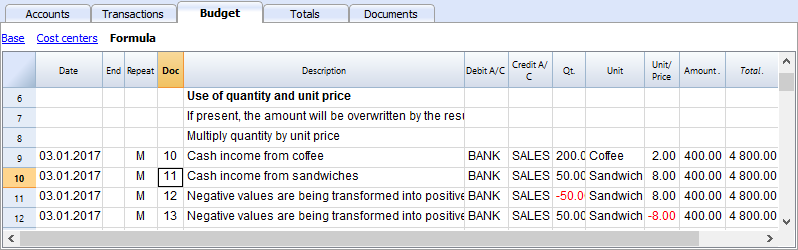In the Formula view you will see the Qt. (quantity) and Unit/Price columns.

• Doc 10. The Qt column. The number (Unit column) of coffees sold in one month at the price of (Unit/price column) and total income from coffee sales (Amount column).
• Values in the Qt and Unit/Price columns can also be entered as a negative number, but the amount will be shown as a positive value.

## Formula in JavaScript language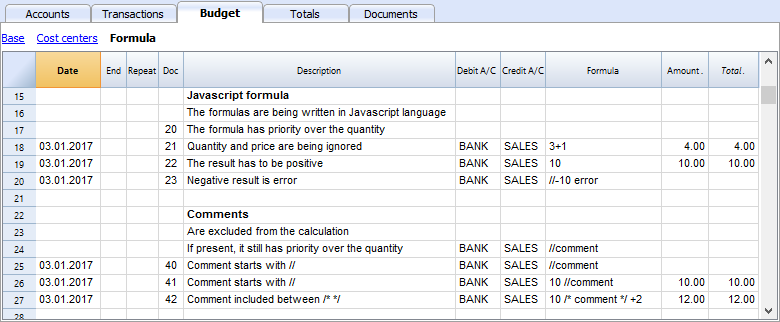The Formula column allows you to enter calculations expressed in JavaScript language.

• If the result of the formula is a number it will be shown as Amount in the respective column.
• Doc. 21+22. You may specify numbers or mathematical expressions.
• Doc 40 e 41. "//" indicates that a comment is entered.
• Doc 42. Text entered between /* e */ are treated as comment and not part of the formula.

## Formula errors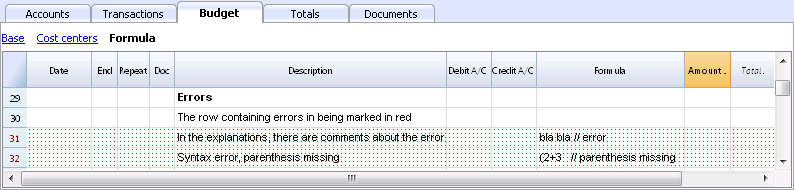In case of errors, when you enter data:

• The row containing the error is highlighted in red.
• An error text is shown in the “message” window.

In order to avoid constant acoustic signals and popping up of error message windows, the errors have been treated as a comment in the example file (they start with "//").

## Semicolon separator ";"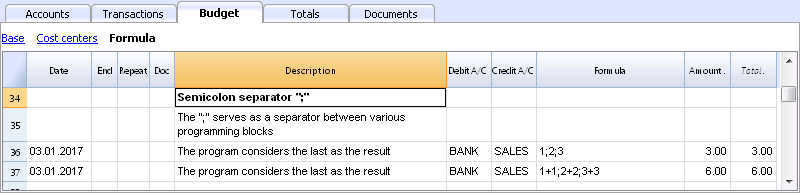JavaScript uses ";" to terminate each line of command. You may concatenate mathematical expressions into one line. The return value will be the result of the last expression.

## Comma separator ","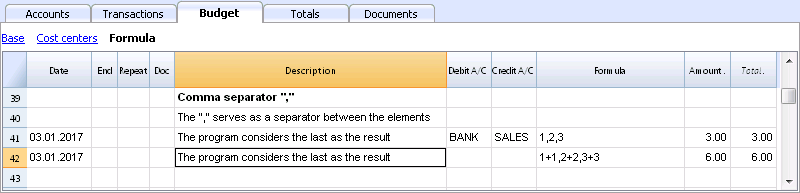In JavaScript, comma is used as a separator between the different expressions in a function. The result value will be the result of the last expression.
Do not use the comma as a decimal separator, because in the formula it will be considered as an expression separator, and therefore numeric values will be truncated.

## Full stop separator for decimals "."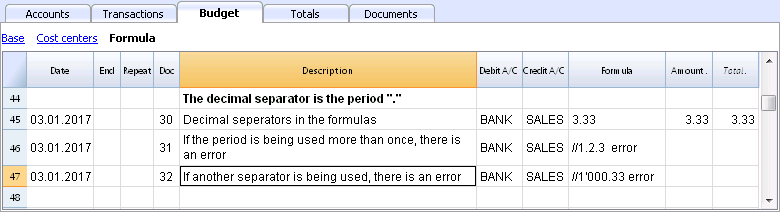JavaScript uses full stop "." as a separator for decimals. Regardless of the settings for decimals in your operating system preferences, full stop will always be used.

## Calculations with simple numbers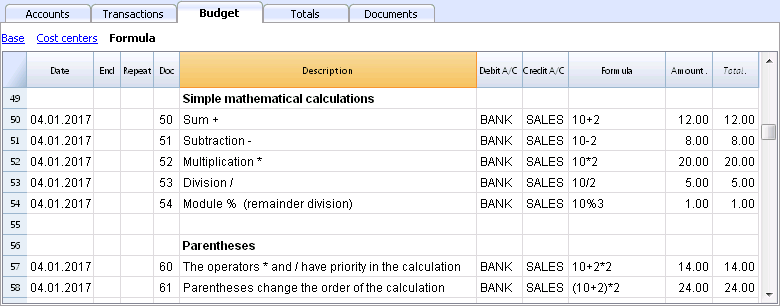You can compound mathematical calculations with simple numbers in the Formula column.

## Texts and variables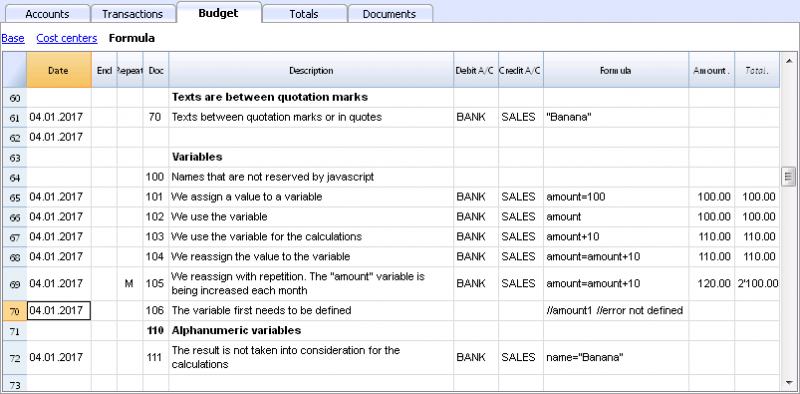• Text is contained between quotation marks, as quotes.
• Variables are the elements that mention the values that have been assigned.
• The name of the variable must start with a letter or an underscore.
• First of all, variables must be defined via a “variablename = 100” assigned punctuation.

Examples:

• Doc 70.
"Banana" between quotation marks is a text
• Doc 101.
A value of 100 is assigned to the variable.
• Doc 102.
The content of the Amount variable will be used.
• Doc 103.
The content of the Amount variable will be used, with an increment of 10.
• Doc 104.
The content of the Amount variable is assigned to the Amount variable with an increment of 10. The Amount variable will be equal to 110.
• Doc 105.
The Formula will be applied on a monthly basis “Repeat=M”
• For the first time 110+10 = 120
• For second time 120 + 10 = 130, and so on.
• Doc 106.
If no comment is entered, an error message will signal that Amount1 has not been defined.
• Doc 110.
The name “Banana” is assigned to the text Variable.

## Controls for if .. then .. else flows

You can use all the conditional expressions of JavaScript. The subject is broad and we suggest you to refer to the documentation on the JavaScript language.
In this regard we only want to point out that in JavaScript:

• The equal sign "=" is used to assign a value to a variable "a = 10"
• On the contrary, for comparison, two successive equal signs are used "==". If (10 == 10) {1};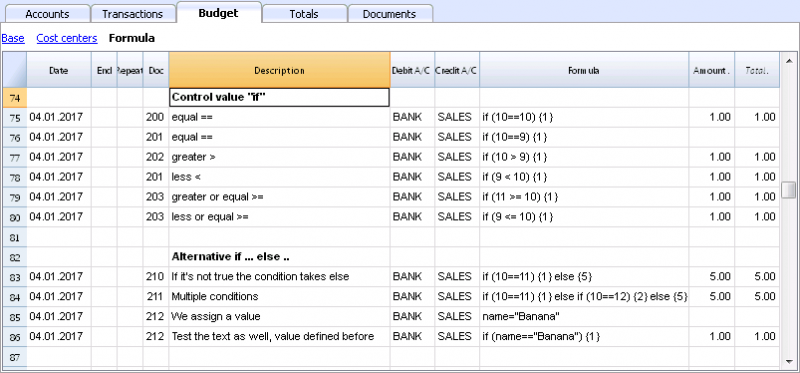• Doc 200-203
If the criteria given in round brackets after the word "if" is true "(10 == 10)", then the Amount column the result of the next expression contained in curly brackets "{1}" will be shown.
If it is not true "(10 == 9)" the amount will be zero.

## Properties and Functions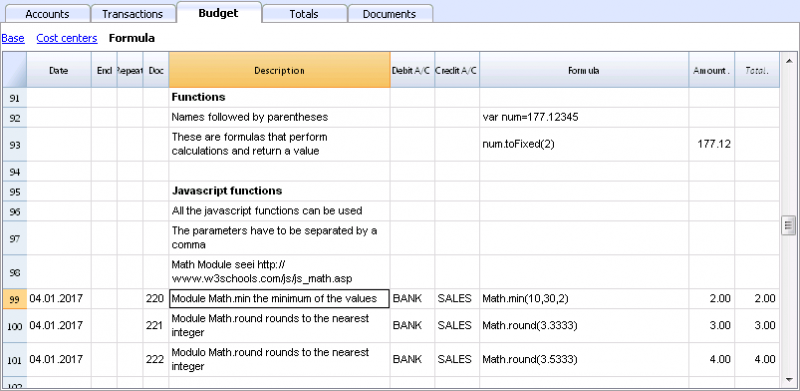Predefined JavaScript functions, such as Module Math.library, can be used (see examples Doc 220-222).

To call up a function, indicate it's name followed by the parameters of the function, indicated between brackets.

• Doc 220.
Math.min(10, 30, 2) will return the minimum value of the values specified between commas.

## “Row object” for current row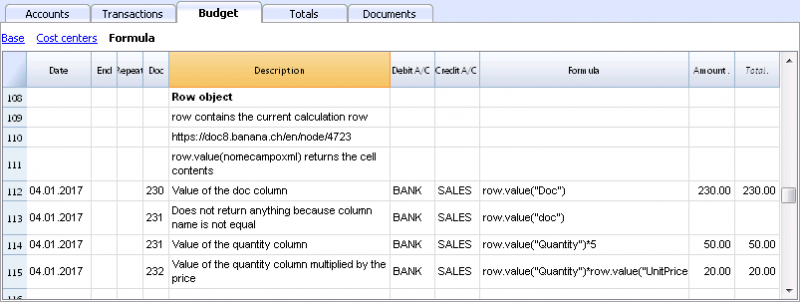Row object is of the Banana.Document.Row type an refers to the current row.

Specifying the name of a column via the Value function, will return the content of that specific column.

## Predefined Debit and Credit functions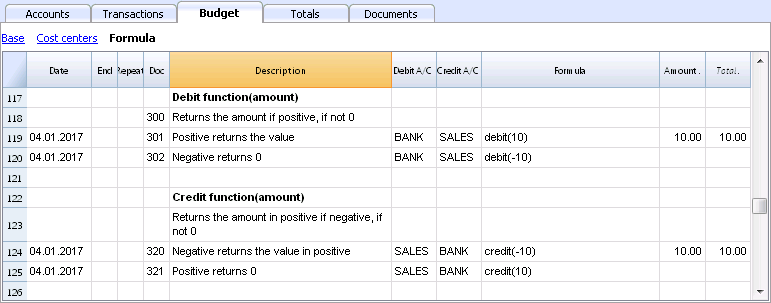Formula only allows for positive values.

• The debit function returns the value entered as a parameter if it is positive.
• The credit function returns the value with as inverted, if the entered value is negative.

## Accounting functions for the entire period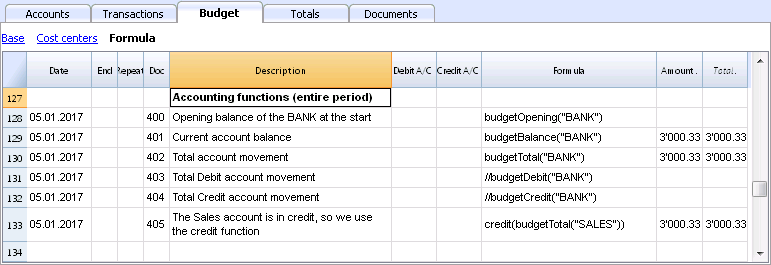Without indication of a defined period, the use of specific Budget functions will return the values relative to the present situation.
Please note and remember, that data in rows is processed sequentially, so there will be no subsequent values to the line being processed.

## Use of accounting functions for a defined period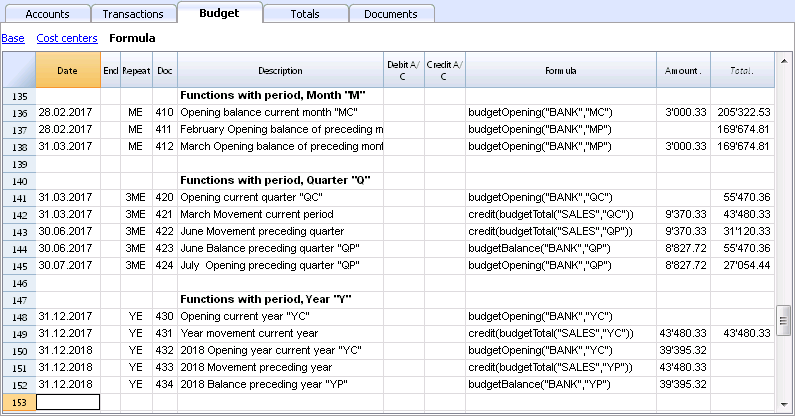Use of accounting functions for a defined period.

The budgetBalance function for the current period will always be the same as the function for the entire period, as the budgetBalance function returns the balance at the end of the period and the last row processed is also the last of the current period.
he budgetOpening and budgetTotal functions, on the contrary, take the initial date into account so it's indication is necessary.

## Interest calculation function

The budgetInterest function calculates interest at entering date, on the indicated account on an actual days count basis (365/365.
When calculating interest for the current month, you must indicate month end as the date.

• Doc 500.
The second parameter represents the interest rate, 5 meaning 5%. If debit interest is due, calculation will be effectuated for the corresponding days.
• Doc 520.
If credit interest is due, calculation will be effectuated for the corresponding days. This will show as a negative towards the BANK account.

## Functions for calculating tax on profit• Doc 600.
The tax on profit is calculated on the movement in the "budgetTotal" period of the final group of the Profit & Loss statement (in this case the 02).
credit (budgetTotal ("Gr = 02", "YC")) * 0.10
• You obtain the group movement in the current year
budgetTotal ("Gr = 02", "YC")
• The credit () function returns the value only if it is negative and converts it to positive.
credit (budgetTotal ("Gr = 02", "YC"))
• The result is multiplied by 0.10 (percentage 10%)
• In the Debit account, the Tax account of the Profit & Loss statement must be indicated.
• In the Credit account, you must indicate the Bank account or the Treasury credit account.

## User-defined Functions

In the Documents table, a "_budget.js" file is added where you can define functions in Javascript that can be called in the formula column.

### Calculating tax on profit with progressive rate

• Doc 610
The profit is first calculated and assigned to the variable
• Doc 611
The profit is passed on to the calcTaxes() function that applies the tax percentage based on the amount and returns the tax amount to be paid.

### Other user-defined functions

• Doc 620
The test () function is called, which is also defined in the budget_js document, which simply returns a value.

## Use of Variables for next calculations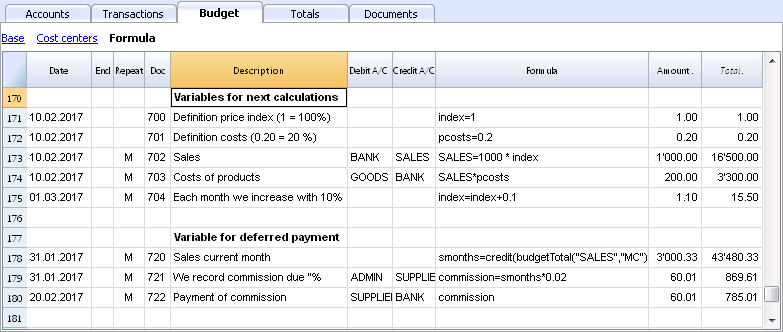Variables are very useful for storing values for next calculations.

• Doc 700-704.
Indexation of sales.
• Doc 700.
Fixation of the index at 1 (100%).
Debit A/C and Credit A/C are not used here, as this row is only used for Definition of the Price index.
• Doc 701.
pscosts variable is used for calculation of acquisition cost (0.2=20%)
• Doc 702.
Sales are a 1000 multiple of the price index value.
• Doc 704.
As per 1st of March the index will be increased by 0.1 (=10%).
As per this date, value of sales will increase in a monthly progression.
• Doc 720-722
Monthly sales figures are assigned to months function. Sales commissions are then calculated

## JrepeatNumber Column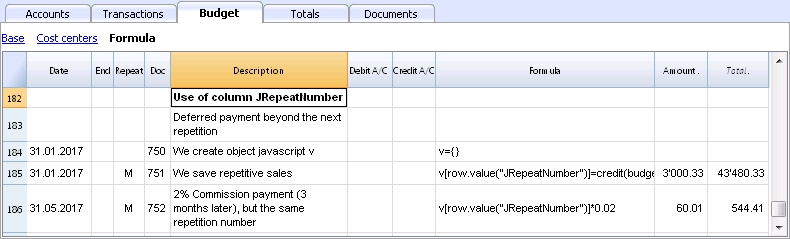When Rows contain a Repeat, insert the Repeat number in the Formula cell as JrepeatNumber.

• First repeat value is 0.
• Second repeat value is 1, and so on.

Use JRepeatNumber when Repeat doesn't allow you to overwrite predefined Variables.
By using JrepeatNumber as a parameter for your object, sequences are registered and can be called at a later stage,

## Free and with no obligation

You can open this template with the Banana Accounting software. If you still don't have Banana Accounting you can download the Free Version. Banana Accounting offers several applications and more than 500 templates to choose from.